# The pot

The pot is a cylinder with a volume of V = 7l and an inner diameter of d = 20cm. Find its depth.

h =  22.3 cm

### Step-by-step explanation: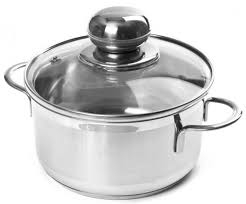Did you find an error or inaccuracy? Feel free to write us. Thank you!Tips to related online calculators
Do you know the volume and unit volume, and want to convert volume units?

#### You need to know the following knowledge to solve this word math problem:

We encourage you to watch this tutorial video on this math problem:

## Related math problems and questions:

• PotCalculate the height of 3 liter pot with shape cylinder with a diameter of 10 cm.
• The potDiameter of the pot 38 cm. The height is 30 cm. How many liters of water can fit in the pot?
• Perimeter of baseThe circumference of the base of the rotating cylinder is same as its height. What is the diameter and height of this cylinder with volume 1 liter?
• Cylinder: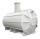Cylinder: calculate the height of a 3 liter cylindrical pot with a diameter of 10 cm.
• Steel tubeThe steel tube has an inner diameter of 4 cm and an outer diameter of 4.8 cm. The density of the steel is 7800 kg/m3. Calculate its length if it weighs 15 kg.
• The hollow cylinderThe hollow cylinder has a height of 70 cm, an outer diameter of 180 cm, and an inner diameter of 120 cm. What is the surface of the body, including the area inside the cavity?
• ConvaHow many liters of water fit into the shape of a cylinder with a bottom diameter 20 cm and a height 45 cm?
• DiameterWhat is the inside diameter of the cylinder container and if half a liter of water reaches a height 15 cm?
• Half-filled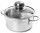A cylindrical pot with a diameter of 24 cm is half-filled with water. How many centimeters will the level rise if we add a liter of water to it?
• Cylinder - hThe cylinder volume is 140 cm3. The base radius is 7 cm. Calculate the height of the cylinder.
• Water levelHow high reaches the water in the cylindrical barell with a diameter of 12 cm if there is a liter of water? Express in cm with an accuracy of 1 decimal place.
• The inflated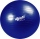The inflated gymnastic ball should have a diameter of 65 cm. How many times do we have to pump air into a fully blown ball with a bicycle inflator to inflate it if the working volume of the inflator is a cylinder with an inner diameter of 2 cm and a heigh
• The largest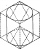We cut the largest possible cylinder from a 20 cm cube. What is the volume of this cylinder?
• Hectoliters of waterThere are 942 hectoliters of water in a cylindrical tank with an inner diameter of 6 m. The water reaches two thirds of the depth of the tank. Calculate its depth.
• Octagonal prism vase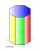0.7 l of water can be poured in an octagonal prism vase. What is the height of the vase, if the bottom has a area of 25 cm square and a thickness of 12 mm?
• The potThe pot is in 1/3 filled with water. Bottom of the pot has an area of ​​329 cm2. How many centimeters rises water level in the pot after add 1.2 liters of water?
• Garden pool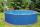Mr. Novak fill garden pool (cylinder; diameter of 200 cm) with 31.4 hl of water. What is the depth of the pool when the water level is 10 cm below the upper edge of the pool?